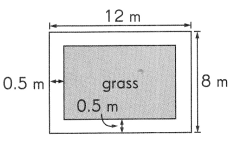This handy Math in Focus Grade 4 Workbook Answer Key End of Year Review detailed solutions for the textbook questions.

Test Prep

Multiple Choice

Fill in the circle next to the correct answer.

Question 1.
The digit 9 in 89.4 stands for _________. (Lesson 7.2)
(A) 9 hundredths
(B) 9 tenths
(C) 9 ones
(D) 9 tens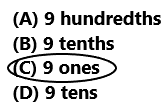So, Option C is correct.

Question 2.
Find 9.50 – 2.63. (Lesson 8.2)
(A) 5.07
(B) 5.73
(C) 6.67
(D) 6.87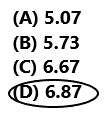9.50 – 2.63 = 6.87
Option C is correct.
Explanation:
Perform subtraction operation on above two numbers. Subtract 2.63 from 9.50 the difference is 6.87. So, draw a circle for option C.

Question 3.
The product of 9 and ____________ is 1,1 07. (Lesson 3.1)
(A) 123
(B) 1,098
(C) 1,116
(D) 9,963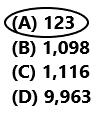The product of 9 and 123 is 1,1 07.
Option C is correct.
Explanation:
Multiply 9 with 123 the product is 1,107. So draw a circle for option C.

Question 4.
The table shows the number of fruits and biscuits a group of students have. Some numbers in the table are missing. Use the information in the table to answer the question. (Lesson 4.1)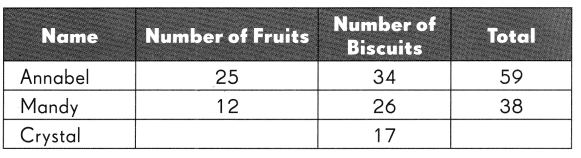The total number of fruits and biscuits is 120. How many fruits does Crystal have?
(A) 6
(B) 23
(C) 37
(D) 97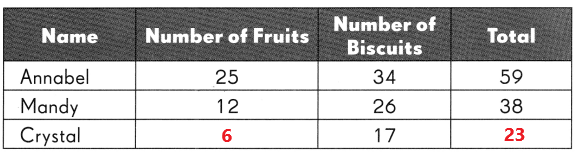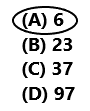The total number of fruits and biscuits is 120.
The total number of fruits and biscuits of Annabel and Mandy  having are calculated by adding 59 and 38.
59 + 38 = 97
Subtract the total fruits and biscuits of Annabel and Mandy from total number of fruits and biscuits.
120 – 97 = 23
Subtract number of biscuits that Crystal having from the total number of fruits and biscuits
23 – 17 = 6
Crystal have 6 fruits.
So, drawn a circle for option A.

Question 5.
The stem-and-leaf plot shows the points scored by Jason in nine basketball games. (Lesson 5.3)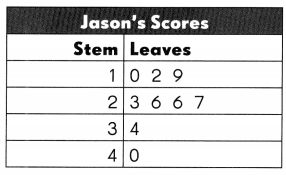What is the outlier of the set of data?
(A) 40
(B) 26
(C) 23
(D) 10

Question 6.
Peter draws one of these number cards from a bag. (Lesson 5.5)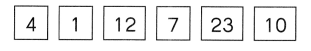What is the probability that he draws a number less than 10?
(A) $$\frac{1}{2}$$
(B) $$\frac{1}{3}$$
(C) $$\frac{1}{4}$$
(D) $$\frac{1}{6}$$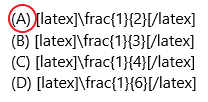Explanation:
Total number of cards are 6.
Number of cards which are having a number less than 10 are 3.
So, the probability the peter draws a number less than 10 = 3/6 = 1/2

Question 7.
Subtract $$\frac{2}{4}$$ from $$\frac{7}{12}$$. Express your answer in simplest form. (Lesson 6.2)
(A) $$\frac{1}{12}$$
(B) $$\frac{2}{15}$$
(C) $$\frac{2}{5}$$
(D) $$\frac{11}{15}$$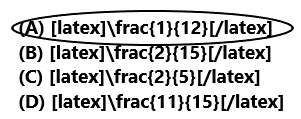7/12 – 2/4 = (7 – 6)/12 = 1/12
So, drawn a circle for Option A.

Question 8.
4$$\frac{3}{5}$$ = ____________ (Lesson 6.3)
(A) $$\frac{12}{5}$$
(B) $$\frac{20}{5}$$
(C) $$\frac{23}{5}$$
(D) $$\frac{43}{5}$$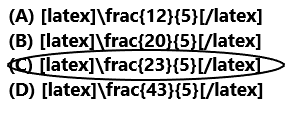4 3/5 = (20 + 3)/5 = 23/5
Option C is correct.

Question 9.
Which of the shaded parts represents $$\frac{4}{5}$$ of a set? (Lesson 6.7)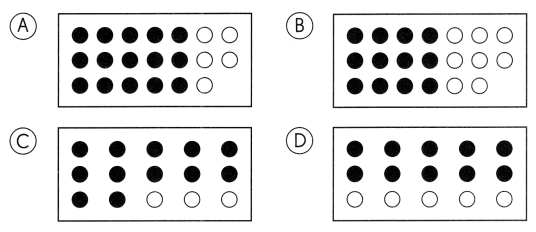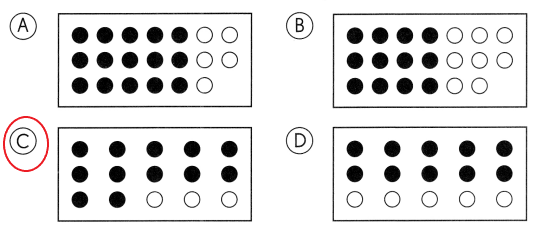Option A:
15 circles are shaded out of 20.
15/20 = 3/4
The simplified form of 15/20 is 3/4.
Option B:
12 circles are shaded out of 20.
12/20 = 3/5
The simplified form of 12/20 is 3/5.
Option C:
12 circles are shaded out of 15.
12/15 = 4/5
The simplified form of 12/15 is 4/5.
Option D:
10 circles are shaded out of 15.
10/15 = 2/3
The simplified form of 10/15 is 2/3.
So, drawn a circle for Option C.

Question 10.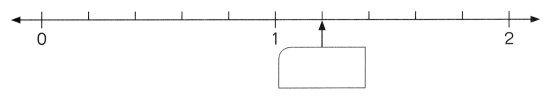The arrow is pointing at __________. (Lesson 7.1)
(A) 0
(B) 1.2
(C) 1.3
(D) 4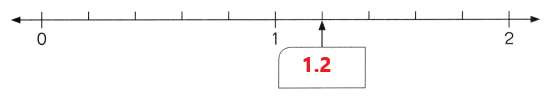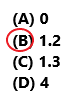The arrow is pointing at 1.2.
So, draw a circle for option B.

Question 11.
Ava’s mass is 45.0 kilograms when rounded to 1 decimal place. What is her least possible mass? (Lesson 7.4)
(A) 45.01 kilograms
(B) 44.95 kilograms
(C) 44.99 kilograms
(D) 44.55 kilograms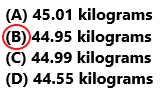Ava’s mass is 45.0 kilograms and it is rounded to 1 decimal place.
The least possible mass is 44.95.
So, draw a circle for option B.

Question 12.
0.55 is not equal to _________. (Lesson 7.5)
(A) $$\frac{11}{20}$$
(B) $$\frac{55}{100}$$
(C) $$\frac{550}{1,000}$$
(D) $$\frac{55}{10}$$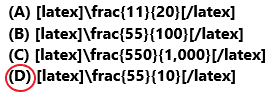Option A:
11/20 = 0.55
Option B:
55/100 = 0.55
Option C:
550/1,000 = 0.55
Option D:
55/10 = 5.5
0.55 is not equal to 55/10.
So, option D is correct.

Question 13.
4.6 – 0.46 is equal to _________. (Lesson 8.2)
(A) 0
(B) 4.14
(C) 4.20
(D) 4.26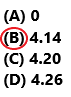4.6 – 0.46 is equal to 4.14.
So, option B is correct.

Question 14.
Which of these angles is an acute angle? (Lesson 9.1)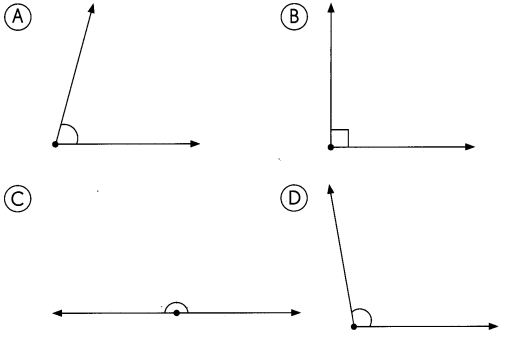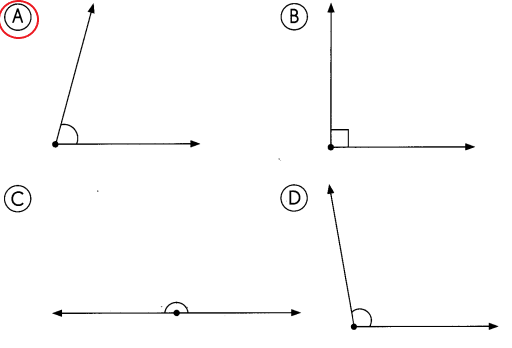Option A is correct.

Question 15.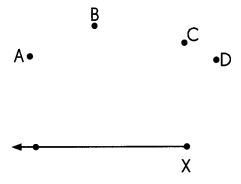Sam needs to draw an angle of 1 25° from point X. He must join point X to point __________. (Lesson 9.2)
(A) A
(B) B
(C) C
(D) D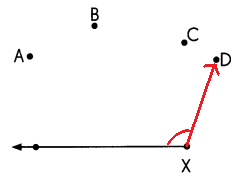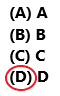Sam drawn an angle of 125° from point X to point D. So, option D is correct.

Question 16.
Refer to the figure to answer Exercises 15 and 16.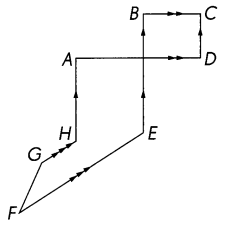Which line segment is perpendicular to $$\overline{\mathrm{AH}}$$? (Lesson 10.1)
(A) HG
(B) BE
(C) FE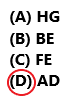The line segment perpendicular to AH is AD.
So, option D is correct.

Question 17.
Which line segment is parallel to $$\overline{\mathrm{CD}}$$? (Lesson 10.2)
(B) GH
(C) BE
(D) FG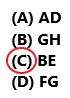The line segment parallel to CD is BE.
So, option C is correct.

Question 18.
In the square below, find the measure of ∠a. (Lesson 11.2)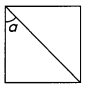(A) 30°
(B) 45°
(C) 60°
(D) 90°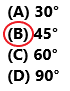The measure of ∠a is 45°.
So, option B is correct.

Question 19.
The perimeter of a rectangle is 24 centimeters. The length of one of its sides is 5 centimeters. What is the area? (Lesson 12.1)
(A) 7 cm2
(B) 14 cm2
(C) 35 cm2
(D) 49 cm2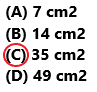The perimeter of a rectangle is 24 centimeters.
The length of one of its sides is 5 centimeters.
Width = ?
Perimeter of a rectangle = 2 (l + w)
24 cm = 2 (5 cm+ w)
24 cm = 10 cm + 2w
24 cm – 10 cm = 2w
14 cm = 2w
7 cm = w
Area of the rectangle = l × w
= 5 cm × 7 cm
= 35 cm2
Area of the rectangle is equal to 35 square centimeters.
So, option C is correct.

Question 20.
All line segments on the figure meet at right angles. Find EF. (Lesson 12.1)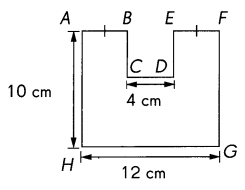(A) 4 cm
(B) 6 cm
(C) 8 cm
(D) 10 cm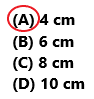From the above figure AB = EF
HG = AB + CD + EF
12 cm = 2 EF + 4 cm
8 cm = 2 EF
4 cm = EF
So, option A is correct.

Question 21.
Which pair of figures are symmetric? (Lesson 13.1)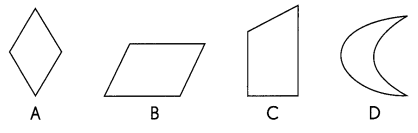(A) A and B
(B) B and C
(C) C and D
(D) D and A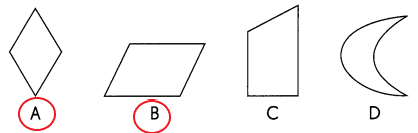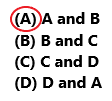The figures A and B are symmetric. So option A is correct.

Question 22.
What is the repeated shape used in the tessellation? (Lesson 14.1)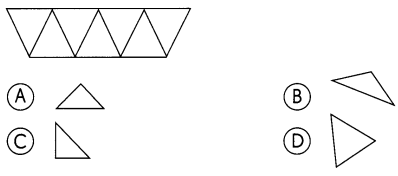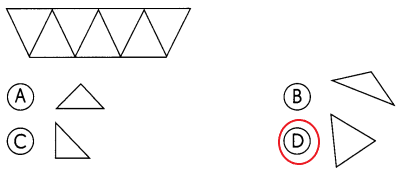The figure in option D is the repeated shape used in the tessellation.

Question 23.
Which of these shapes has rotational symmetry? (Lesson 13.2)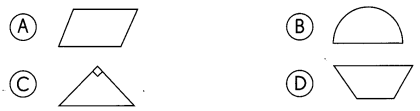Question 24.
This shape can be tessellated by ___________. (Lesson 14.2)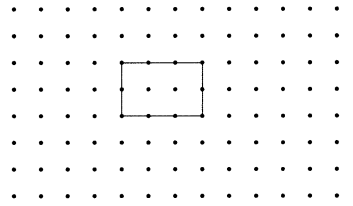(A) sliding
(B) rotation
(C) flipping
(D) All of the above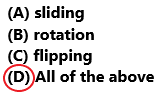The shape can be tessellated by sliding, rotating, and flipping. So, option D is correct.

Question 25.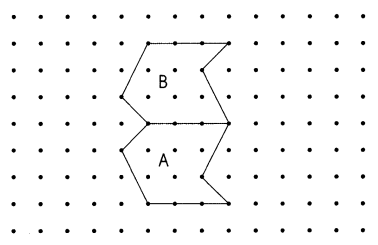From position A to B, the unit shape has been ___________
(A) slid
(B) rotated
(C) flipped
(D) none of the above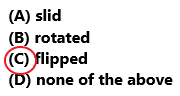From position A to B, the unit shape has been flipped. So, option C is correct.

Question 26.
I am a number between 30 and 50. I am a multiple of 8. My greatest common factor with 25 is 5. What number am I? (Lessons 2.2 and 2.3)
I am a number between 30 and 50.
I am a multiple of 8.
My greatest common factor with 25 is 5.
5 x 8 = 40
The number is 40.

Question 27.
The table shows the number of marbles Anthony and Michelle have. Complete the table and answer the questions. (Lesson 4.1)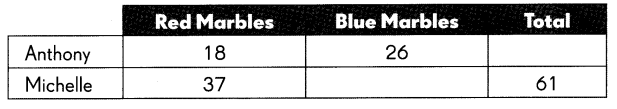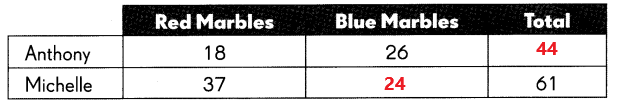a. What was the total number of red marbles?
The number of red marbles does Anthony have is 18.
The number of red marbles does Michelle have is 37.
18 + 37 = 55
The total number of red marbles are 55.

b. What fraction of the total number of marbles were blue?
The total number of red marbles and blue marbles are 105.
44 + 61 = 105
The total number of red marbles are 55.
18 + 37 = 55
The fraction form of the total number of blue marbles.
55/105 = 10/21

Question 28.
The graph shows the amount of water used by the residents of an apartment block over a morning. (Lesson 4.3)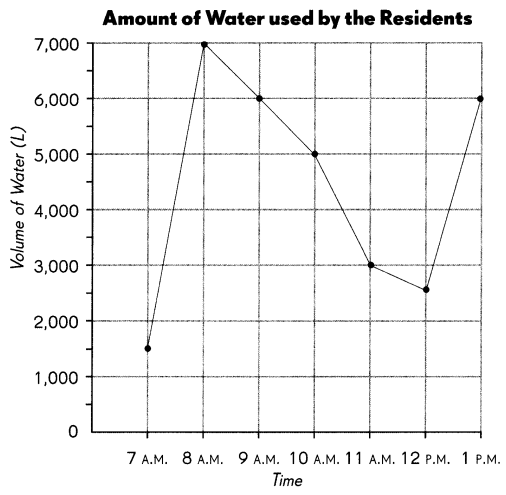a. At which two times was the same amount of water used?
The same amount of water used at 9 A.M and 1 P.M

b. At what time was the amount of water used twice that used at noon?
At 12 P.M the volume of water used is 2,500.
2 x 2,500 = 5,000
At 10 A.M the amount of water used twice that used at noon.

Question 29.
A bag has 5 pink balls, 8 yellow balls, and 4 blue balls. What is the probability of drawing a pink ball from the bag? (Lesson 5.5)
5/(5 + 8 + 4) = 5/17
The probability of drawing a pink ball from the bag is 5/17.

Question 30.
What is $$\frac{7}{12}$$ – $$\frac{2}{6}$$? Express your answer in simplest form. (Lesson 6.2)
7/12 – 2/6 = (7 – 4)/12 = 3/12 = 1/4
The simplest form of 7/12 – 2/6 is 1/4.

Question 31.
Express $$\frac{30}{7}$$ as a mixed number. (Lesson 6.5)
The mixed number for 30/7 is 4 2/7.

Question 32.
Find the difference between $$\frac{5}{8}$$ and 3. (Lesson 6.6)
3 – (5/8) = (24 – 5)/8 = 19/8
The difference between 5/8 and 3 is 19/8.

Question 33.
How many grey squares must be replaced by white squares so that $$\frac{2}{3}$$ of the total number of squares are grey? (Lesson 6.7)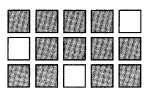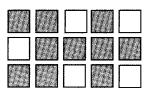Total number of squares = 15
Total number of grey squares = 12
If 2/3 of the total number of squares should be grey then number of grey squares should be = 2/3 x 15 = 10
As total number of grey squares are currently 12. S0, the number of squares to be whitened = 12 – 10 = 2

Question 34.
What is the number in the box? (Lesson 7.2)
6.34 = 6 + 0.3 + ___________
6.34 = 6 + 0.3 + 0.04

Question 35.
Li Li is 1.85 meters tall. Round her height to the nearest tenth of a meter. (Lesson 7.4)
Round her height to the nearest tenth of a meter 1.9.

Question 36.
Express 5$$\frac{6}{25}$$ as a decimal. (Lesson 7.5)
5 6/25 = (125 + 6)/25 = 131/25 = 5.24

Question 37.
Draw and label a line segment BC such that the measure of angle ABC is 167°. Line segment AB is given. (Lesson 9.2)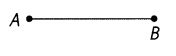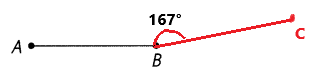Drawn and labeled a line segment BC such that the measure of angle ABC is 167°.

Question 38.
Draw a line segment perpendicular to AB through point O. (Lesson 10.1)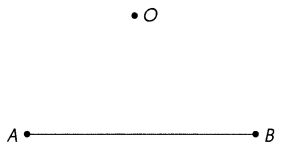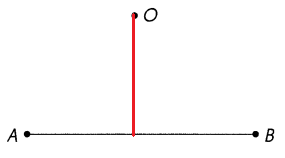Drawn a line segment perpendicular to AB through point O as we can observe in the above image.

Question 39.
Draw a line parallel to $$\overleftrightarrow{C D}$$ passing through point X. (Lesson 10.2)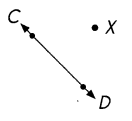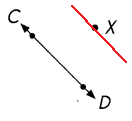Drawn a line parallel to CD passing through point X.

Question 40.
AB is a vertical line segment and BC is a horizontal line segment. Find the measure of ∠ABC. (Lesson 10.3)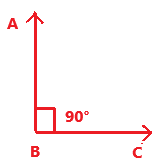Explanation:
AB is a vertical line segment and BC is a horizontal line segment. The measure of ∠ABC is 90°.

Question 41.
Look at the figure below to answer the question. (Lesson 12.3)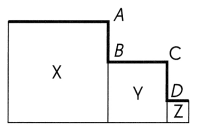X, Y, and Z are squares. The length of each side of X is 5 centimeters and the length of each side of Y is 3 centimeters. AB = CD. Find the total length of the thick lines in the figure.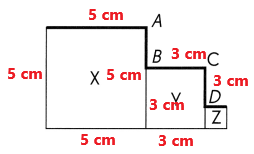In the above image we can observe X, Y, and Z are squares.
The length of each side of X is 5 centimeters and the length of each side of Y is 3 centimeters.
AB = CD.
AB = 5 cm – 3 cm
AB = 2 cm
The total length of the thick line in the figure = 5 + 2 + 3 + 2 + 1 = 13 cm

Question 42.
Shade some squares and half-squares to make a symmetric pattern in the figure. (Lesson 13.3)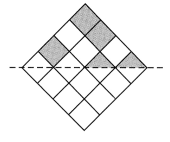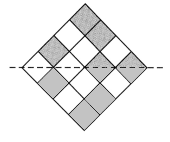Question 43.
In the tessellation below, the unit shape is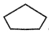. Extend the tessellation in the space provided by adding four more unit shapes. (Lesson 14.2)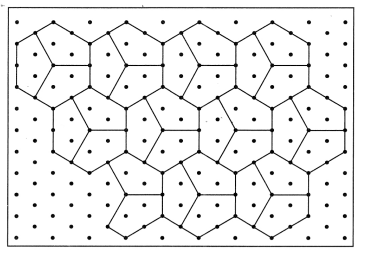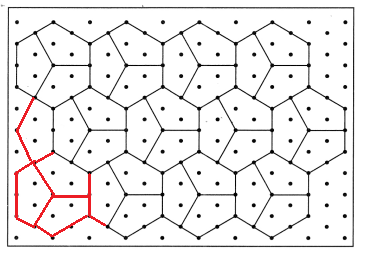By adding four more unit shapes the tessellation is extended in the space provided above.

Question 44.
Complete the tessellation by adding three more unit shapes. (Lesson 14.2)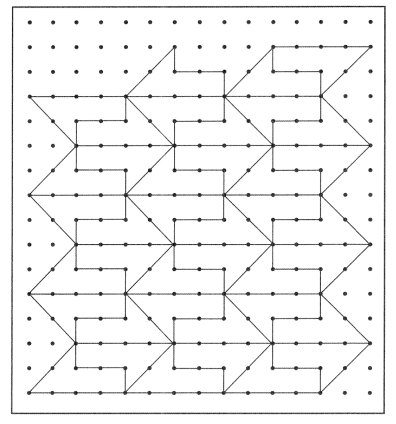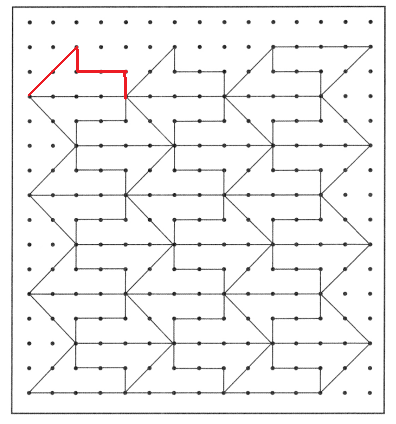Question 45.
Complete the figure so that it has rotational symmetry about point O. (Lesson 13.3)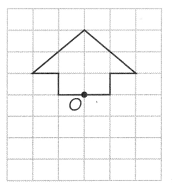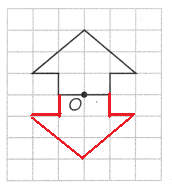The above figure has rotational symmetry at point O.

Question 46.
a. Does the word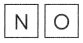have rotational symmetry? (Lesson 13.3)
No, The word ‘NO’ doesn’t have a rotational symmetry.

b. Fill in the box with a letter so that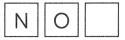will have rotational symmetry. (Lesson 13.3)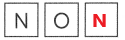The letter NON have rotational symmetry.

Extended Response

Question 47.
Jane used $$\frac{1}{4}$$ of the flour to make biscuits. She used $$\frac{1}{2}$$ of the flour to bake a cake. What fraction of the flour was left?
Jane used 1/4 of the flour to make biscuits.
She used 1/2 of the flour to bake a cake.
The fraction of flour left = 1/2 – 1/4 = 1/4

Question 48.
Mr. Lim has some savings. If he gives $40 to one brother, he will have$6,145 left. But he decides to give all his savings to his 5 brothers equally. How much will each brother get?
$40 +$6,145 = $6,185 He decides to give all his savings to his 5 brothers equally.$6,185/5 = $1237 Each brother will get$1237.

Question 49.
Rita bought fabric and ribbon from a store. The ribbon cost $18.50. Rita paid the cashier$50.00 and received a change of $5.25. How much did the fabric cost? Answer: The ribbon cost$18.50.
The fabric cost = ?
Rita paid the cashier $50.00 and received a change of$5.25.
Rita received change from cashier = Amount paid to the cashier – Ribbon cost – Fabric cost
$5.25 =$50.00 – $18.50 – Fabric cost Fabric cost =$50.00 – $18.50 –$5.25
Fabric cost = \$26.25

Question 50.
The area of a rectangle is 98 square centimeters, and its width is 7 centimeters. Find the length.
The area of a rectangle is 98 square centimeters.
Width = 7 cm
Length = ?
Area of the rectangle = l × w
98 cm2= l × 7 cm
14 cm = l
The length of a rectangle is 14 cm.

Question 51.
Richard planted some grass on a rectangular plot of land which measures 1 2 meters by 8 meters. He left a margin of 0.5 meters around the grass, as shown in the figure below. Find the area of land covered by grass. (Lesson 12.4)